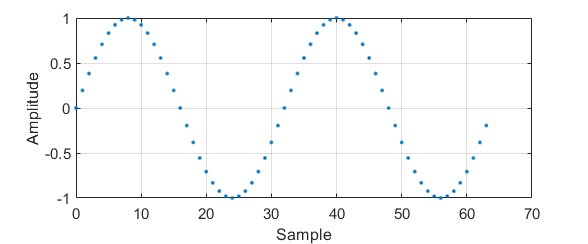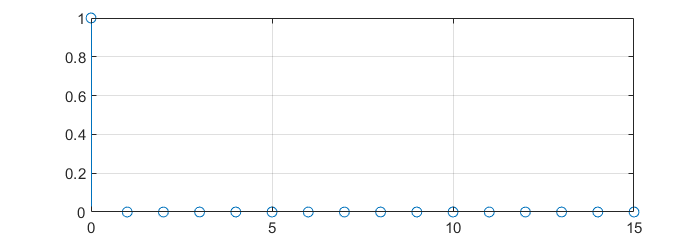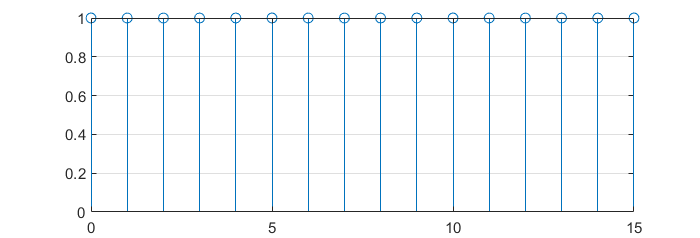## Frequency aliasing (or folding)

In the following Python script it is shown how the aliasing effect (having signals whose frequency is beyond half of the sampling frequency, i.e., $$\frac{f_s}{2}$$) can be intuitively understood as a folding of the frequencies outside the $$\left[0, \frac{f_s}{2}\right]$$ range.

In the script, the sampling frequency is 10 kHz. Then, 3 sinusoids of frequency $$f_{s1} = 500~Hz$$, $$f_{s2} = 6~kHz$$ and $$f_{s3} = 11~kHz$$ are generated. The FFT of the sum of s1, s2 and s3 is computed and plotted. Given that the Nyquist frequency in the system is 5 kHz, it can be seen on the FFT plot how the frequencies wrap around $$\frac{f_s}{2}$$ and 0.

Just as a reminder, the way the X-axis on the FFT is explained in FFT resolution.

import numpy as np
import numpy.fft as fft
import matplotlib.pyplot as plt

# Define sampling frequency
fs = 10e3
Ts = 1/fs

# Define time array
n_periods = 1000
total_time = 1/fs * n_periods
t = np.arange(0, total_time, Ts)

# %% Define signal 1
f1 = 500
s1 = np.sin(2*np.pi*f1*t)

# %% Define signal 2
# Since fs = 5_000, f2 will fold to 4_000 Hz bin
f2 = 6_000
s2 = 0.5 * np.sin(2*np.pi*f2*t)

# %% Define signal 3
# Since fs = 5_000, f3 will fold first on 5_000 Hz and then on 0 again. Therefore, f3 will be shown at 1_000 Hz bin
f3 = 11_000
s3 = 0.25 * np.sin(2*np.pi*f3*t)

# %% Define signal as a sum of s1, s2 and s3
s = s1 + s2 + s3

# %% Compute FFT
# Total number of samples
N_samples = len(s)
print(f"Number of samples: {N_samples}")
# Floor in case division is not integer
half_N_samples = int(np.floor(N_samples/2))
# Define frequency bin width
delta_f = fs/N_samples

# Compute frequency bins until Nyquist frequency
freq_bins = np.arange(0, fs/2, delta_f)

Y = fft.fft(s)

plt.figure(figsize=(8,6), dpi=300)

# Plot only half of the FFT result. Plot absolute value and scale it to represent amplitude
plt.plot(freq_bins, 2.0 / N_samples * np.abs(Y)[:half_N_samples])
plt.grid(True, which='both')
plt.minorticks_on()
plt.title('Signal FFT')
plt.xlabel('Frequency (Hz)')
plt.ylabel('Amplitude')
plt.show()## DFT as a change of basis

DFT can be thought as a simple change of basis. In the time domain, every sample of a signal represents its amplitude at that very instant.

$y\left[n\right] = \sin{\left( \frac{2\pi k }{N} n\right)}$$k = 2, N = 64$

Nevertheless, when applying a transformation such as the DFT, every sample means something different.

The DFT is computed as the scalar product between the signal and a orthogonal base. This base is as follows:

$\{\boldsymbol{w}^{(k)}\}_{\{k=0,1,…,N-1\}}$

$w_n = e^{j \frac{2\pi}{N}nk}$

If some of these base vectors are plotted with the imaginary part in the Y axe and the real part in the X axe:## DFT calculation

The DFT of a signal can be computed then, applying a change of basis over the desired signal.

$X\left[k\right] = \sum_{n=0}^{N-1} x\left[n\right] e^{-j\frac{2\pi}{N}nk}, \text{ } k = 0,1,…,N-1$

### DFT of a $$\delta\left[n\right]$$

$x\left[n\right] = \delta[n] = \left\{\begin{matrix} 1 & n = 0\\ 0 & otherwise \end{matrix}\right.$$X\left[k\right] = \sum_{n=0}^{N-1}{\delta\left[n\right] e^{-j\frac{2\pi}{N}nk}}$

$X\left[k\right] = 1 \cdot e^{-j\frac{2\pi}{N}\cdot 0 \cdot k} = 1$

###DFT of a cosine

$x\left[n\right] = 4 \cos{\left(\frac{2\pi}{4}n\right)} = 4 \cos{\left(\frac{2\pi 4}{16}n\right)} = \frac{4}{2} \left[e^{ \frac{2\pi}{16} 4n} + e^{-j \frac{2\pi}{16} 4n} \right]$

[To be finished]

## Basis in a vector space

A vector space basis is the skeleton from which a vector space is built. It allows to decompose any signal into a linear combination of simple building blocks, namely, the basis vectors. The Fourier Transform is just a change of basis.

A vector basis is the linear combination of a set of vector that can write any vector of the space.

$w^{(k)} \leftarrow \text{basis}$

The canonical basis in $$\mathbb{R}^2$$ are:

$$e^{(0)} = [1, 0]^T \ \ e^{(1) } = [0,1]^T$$

Nevertheless, there are more basis for $$\mathbb{R}^2$$:

$$e^{(0)} = [1, 0]^T \ \ e^{(1) } = [1,1]^T$$

This former basis is not linearly independent as information of $$e^{(0)}$$ is inside $$e^{(1)}$$.

## Formal definition

H is a vector space.

W is a set of vectors from H such that $$W = \left\{ w^{(k)} \right\}$$

W is a basis of H if:

1. We can write $$\forall x \in H$$: $$x = \sum_{k=0}^{K-1} \alpha_k w^{(k)}, \ \ \alpha_k \in \mathbb{C}$$
2. $$\alpha_k$$ are unique, namely, there is linear independence in the basis, as a given point can only be expressed in a unique combination of the basis.

Orthogonal basis are those which inner product returns 0:

$$\left \langle w^{(k)}, w^{(n)} \right \rangle = 0, \ \ \text{for } k \neq n$$

In addition, if the self inner product of every basis element return 1, the basis are orthonormal.

## How to change the basis?

An element in the vector space can be represented with a new basis computing the projection of the current basis in the new basis. If $$x$$ is a vector element and is represented with the vector basis $$w^{(K)}$$ with the coefficients $$a_k$$, it can also be represented as a linear combination of the basis $$v^{(k)}$$ with the coefficients $$\beta_k$$. In a mathematical notation:

$x = \sum_{k=0}^{K-1} \alpha_k w^{(k)} = \sum_{k=0}^{K-1} \beta_k v^{(k)}$

If $$\left\{ v^{(k)} \right\}$$ is orthonormal, the new coefficients $$\beta_k$$ can be computed as a linear combination of the previous coefficients and the projection of the new basis over the original one:

$\beta_h = \left \langle v^{(h)}, x \right \rangle = \left \langle v^{(h)}, \sum_{k=0}^{K-1} \alpha_k w^{(k)} \right \rangle = \sum_{k=0}^{K-1} \alpha_k \left\langle v^{(h)}, w^{(k)} \right \rangle$

This operation can also be represented in a matrix form as follows:

$\beta_h = \begin{bmatrix} c_{00} & c_{01} & \cdots & c_{0\left(K-1 \right )}\\ & & \vdots & \\ c_{\left(K-1 \right )0} & c_{\left(K-1 \right )01} & \cdots & c_{\left(K-1 \right )\left(K-1 \right )} \end{bmatrix}\begin{bmatrix} \alpha_0 \\ \vdots \\ \alpha_{K-1} \end{bmatrix}$

This operation is widely used in algebra. A well-known example of a change of basis could be the Discrete Fourier Transform (DFT).

## Inner product in vector space

The inner product is an operation that measures the similarity between vectors.  In a general way, the inner product could be defined as an operation of 2 operands, which are elements of a vector space. The result is a scalar in the set of the complex numbers:

$\left \langle \cdot, \cdot \right \rangle : V \times V \rightarrow \mathbb{C}$

## Formal properties

For $$x, y, z \in V$$ and $$\alpha \in \mathbb{C}$$, the inner product must fulfill the following rules:

To be distributive to vector addition:

$$\left \langle x+y, z \right \rangle = \left \langle x, z \right \rangle + \left \langle y, z \right \rangle$$

Conmutative with conjugate (applies when vectors are complex):

$$\left \langle x,y \right \rangle = \left \langle y, x \right \rangle^*$$

Distributive respect scalar multiplication:

$$\left \langle \alpha x, y \right \rangle = \alpha^* \left \langle x, u \right \rangle$$

$$\left \langle x, \alpha y \right \rangle = \alpha \left \langle x, u \right \rangle$$

The self inner product must be necessarily a real number:

$$\left \langle x, x \right \rangle \geq 0$$

The self inner product can be zero only when the element is the null element:

$$\left \langle x,x \right \rangle = 0 \Leftrightarrow x = 0$$

## Inner product in $$\mathbb{R}^2$$

The inner product in $$\mathbb{R}^2$$ is defined as follows:

$$\left \langle x, y \right \rangle = x_0 y_0 + x_1 y_1$$

In self inner product represents the squared norm of the vector:

$$\left \langle x, x \right \rangle = x^2_0 + y^2_0 = \left \| x \right \|^2$$

## Inner product in finite length signals

In this case, the inner product is defined as:

$\left \langle x ,y \right \rangle = \sum_{n= 0}^{N-1} x^*[n] y[n]$

## Properties of vector spaces

Vector spaces must meet the following rules:

$$x + y = y + x$$

$$(x+y)+z = x + (y + z)$$

Scalar multiplication to be distributive with respect to vector addition:

$$\alpha\left(x + y \right) = \alpha x + \alpha y$$

Scalar multiplication to be distributive with respect to vector the addition of field scalars:

$$\left( \alpha + \beta \right) x = \alpha x + \beta y$$

Scalar multiplication to be associative:

$$\alpha\left(\beta x \right) = \left(\alpha \beta \right) x$$

It must exist a null element:

$$\exists 0 \in V \ \ | \ \ x + 0 = 0 + x = x$$

It must exist an inverse element for every element in the vector space:

$$\forall x \in V \exists (-x)\ \ | \ \ x + (-x) = 0$$

## Linear operator

A linear operator must meet the following conditions:
$S\{\alpha x[n]\} = \alpha S\{x[n]\}$
$S\{x[n] + y[n] \} = S\{x[n] \} + S\{y[n] \}$

## Energy and power of a signal

$E_x = \sum_{n=-\infty}^{+\infty} \left| x\left[ n \right] \right|^2$
$P_x = \lim_{N\to\infty} \frac{1}{2N + 1} \sum_{n = -N}^{N} \left| x[n] \right|^2$

## Not all sinusoids are periodic in discrete time

For a signal to be periodic, it must fulfill the following condition:

$x[n] = x[n+M]$
where $$M \in \mathbb{Z}$$.

If $$x[n] = e^{j\left(\omega n + \phi\right)}$$, then:

$e^{j\left(\omega n + \phi\right)} = e^{j\left(\omega \left(n+M\right) + \phi\right)}$

$e^{j\omega n } e^{j\phi} = e^{j\omega n }e^{j\omega M} e^{j\phi}$

$1 =e^{j\omega M}$

For $$e^{j \omega M}$$ to be 1, the angle must be multiple of $$2\pi$$. Then:

$\omega M = 2\pi N$

where $$N \in \mathbb{Z}$$ and $$2 \pi N$$ represents any integer multiple of $$2\pi$$.
Then, the frequency must meet:
$\omega = \frac{2\pi N}{M}$

This is, $$\omega$$ must be a rational multiple of $$2\pi$$.

What does this mean?

Basically, that a periodic signal can’t take any arbitrary frequency in discrete time.

## Resolución FFT

$\Delta f = \frac{F_s}{N_{samples}}$

Si por ejemplo, $$F_s = 5~GSa/s = 5\cdot10^9~Sa/s$$ y $$N_{samples} = 25000$$, la resolución frecuencial es de:

$\Delta f = \frac{5 \cdot 10^9}{25000} = 200~kHz$